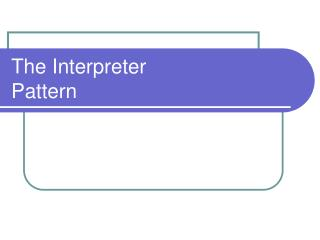# The Interpreter Pattern - PowerPoint PPT PresentationDownload PresentationThe Interpreter Pattern

The Interpreter PatternDownload Presentation## The Interpreter Pattern

- - - - - - - - - - - - - - - - - - - - - - - - - - - E N D - - - - - - - - - - - - - - - - - - - - - - - - - - -
##### Presentation Transcript

1. The InterpreterPattern

2. Defining the Interpreter • Intent • Given a language, define a representation for its grammar along with an interpreter that uses the representation to interpret sentences in the language.

3. Pieces of the Interpreter • Abstract Expression • Terminal Expressions • NonTerminal Expression • Context • Hold global information for the interpreter • Client • Builds the Abstract Syntax Tree • Invokes Interpret on the tree

4. Flow of the Interpreter • Build the Abstract Syntax Tree • Interpret the tree in the given Context • Each non-terminal node will interpret its children and return a result from that. • Terminal nodes return actual values for non-terminal nodes to use. • The context serves as a global data for interpreting the entire tree

5. Example Language: Arithmetic • Grammar • Equation ::= Variable | Integer | Plus | Minus | Times | Divide | Mod | Negate | ‘(‘ Eq ‘) • Terminal equations • Variable::= ‘a’ | ‘b’ | ‘c’ …. |”ab” | … • Integer::= 1 | 2 | 3 | 4 | 5 | 6 | … • Non-Terminal equations • Plus::= Eq ‘+’ Eq Minus::= Eq ‘-’ Eq Times::= Eq ’*’ Eq Divide::= Eq ‘-’ Eq Mod::= Eq ‘%’ Eq …Negate:: ‘-’ Eq

6. Abstract Syntax Tree Example • 5 * 2 + B

7. Consequences • Easily extensible grammar • Implementing grammar is easy • Complex grammar is difficult to manage • Can easily add new ways to interpret expressions through the Visitor.

8. Related Patterns • Composite • The Composite pattern is essential for representing the AST • Interator • Can be useful for traveling the AST • Visitor • Essential for implementing multiple ways of interpreting the AST • Flyweight • Especially useful when terminal symbols are used repetitively

9. Common uses • Widely used in compilers that are implemented with an OO language • Any case where you use the Composite Pattern to represent a language